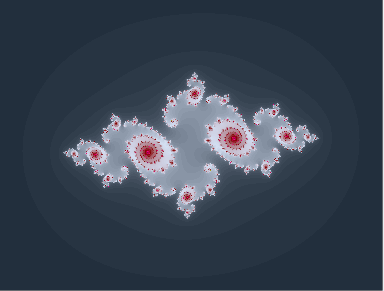## The Theory

All images of this edition show magnifications cut out of the edge of the mandelbrot set. This set is named after the mathematician Benoit B. Mandelbrot, who discovered their fractal and self replicating structure and therefore founded the modern fractal geometry.

### The Magic Formula

It is mathematically described as the connected set of points c within the complex plane, where the sequence of

|Zn| with Zn+1=Zn2+c and Z0=(0,0)

stays bounded for infinite growing n. The pair (x,y) describes the complex number x+iy, where i2 = -1. The exclusion of points from the set is based on a property of the iteration formula which says that |Zn| is infinitely growing (or escaping from the origin) if the point lies outside a circle of a definite radius around the origin. A typical value for this criteria is 2. If such a value is reached during the iteration, it is sure that the iteration values are unbounded and the starting value for the iteration definitly does not belong to the mandelbrot set.

Unfortunately this way it is only possible to detect that a point does not belong to the set but not that it does. For all points not belonging to the set there is an n for which this criterion matches, but n might be of any height.

Basically the closer you approach to the edge of the mandelbrot set the higher is n for points not belonging to the set. This means that more iteration steps have to be done to be able to resolve details of the edge.

The whole mandelbrot set looks like a man made of spheres of apples - therefore it is often called the apple man (Apfelmaennchen in german). This apple man is the dominant structure of the mandelbrot set that can be found in all magnification levels due to the property of self replication. Whatever structure of the edge of the mandelbrot set is visible, this constant is always there, may be you just have to increase the magnification. Therefore in many pictures shown on this site you can find this apple man and others you don't, but believe me, it is there - you just have to invest in a magnifying glass. Some of the best pictures cover areas where the apple man is not directly visible and other structures of the edge get visible, because the standard structure is hidden in the deepness.### Mandelbrot vs. Julia Set

The same formula can be used to calculate Julia sets. A Julia set can be calculated for every point of a mandelbot image. Here the value c is fixed for the whole set. Instead of c the starting value of the iteration Z0 is varied according the coordinates of the image. The images presented by this site are all mandelbrot pictures, although some images may look like a typical julia set.

The difference is that for magnifications of the edge of the mandelbrot set there are always outgoing filaments, because the whole set is connected. So you'll never find structures like the Julia set above, that are fully enclosed by the same iteration depth or color.